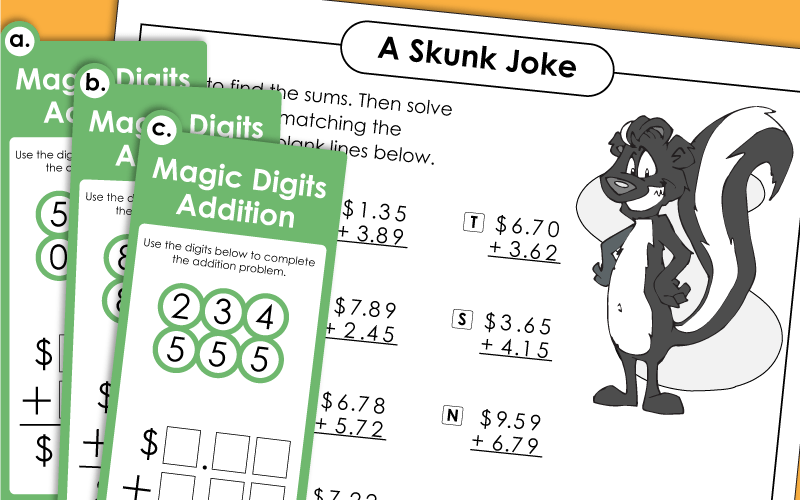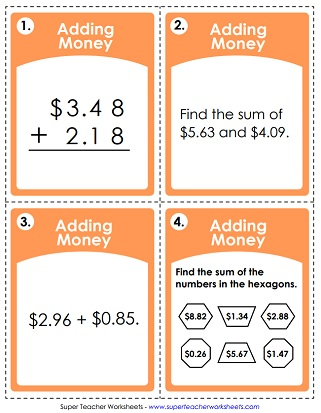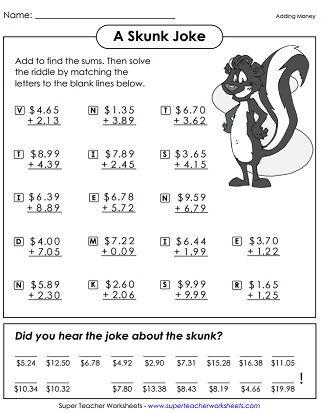These worksheets have money addition problems. Problems are written vertically and horizontally. Includes word problems, and a fun math riddle worksheet.Add to find the total cost of the bakery items pictured.
Solve addition problems with money addends. Then use the sums to decode a funny joke about a skunk.
At the top of this page are money amounts with shapes around them. Add the numbers in similar shapes together. For example: Find the sum of the numbers in the triangles.
Practice adding money with this worksheet. It includes several styles of math problems, such as word problems and fill-in-the-blanks.
Add the money amounts to determine how much you'll spend on your family members at the Secret Santa Market.
Addition problems with money amounts; Winter snowmen pictures.
Add up the money amounts to find the total cost of the items.
These math problems were already solved, but there are mistakes. Explain how the problems were incorrectly solved. Then calculate the correct sums.
The money addition problems on this worksheet are each written horizontally. Students will need to rewrite them vertically to solve.
Add the money amounts to find the sums. Then cut out the picture puzzle pieces. Match each numbered (sum) piece to the correct problem. The completed picture shows a hot air balloon.
Choose a money amount from each wheel. Then calculate the sum.

This file has 30 task cards with vertical and horizontal money addition problems.
In this game, students will work with task cards that have digits. They must arrange the digits to complete the addends in the addition problem.

Find the missing value for each bar model. Then write an addition and subtraction number sentence for each.
In this math activity, students will find the missing value for each bar model, then they will write an addition and subtraction number sentence for each one.

Add 2, 3, 4, and 5-digit numbers. Also includes addition worksheets with fractions, mixed numbers, and decimals.

Subtracting Money

## Worksheet ImagesMy Account
Site Information